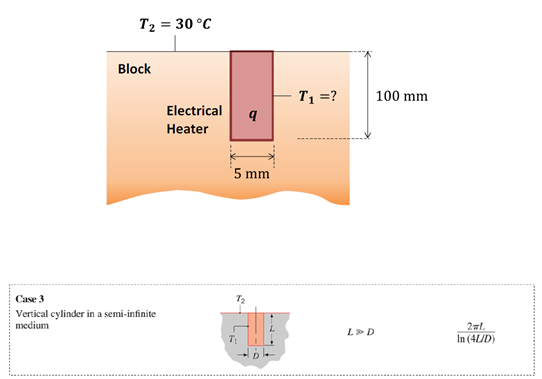## Electrical Heater Rod

Consider an electrical heater rod that is 5 mm in diameter by 100 mm long, and is installed normal to the surface of a different material that has a 5 W/m∙K thermal conductivity. If the block’s surface temperature is 30°C, and the rod dissipates 50 W, calculate the heater’s temperature.Hint
Heat loss can be expressed as:
$$q=kS(T_{1}-T_{2})$$$where $$k$$ is the thermal conductivity, $$S$$ is the shape factor, and $$T$$ is the temperature. Hint 2 Solve for $$T_1$$ : $$T_{1}=T_{2}+\frac{q}{kS}$$$
Heat loss can be expressed as:
$$q=kS(T_{1}-T_{2})\rightarrow T_{1}=T_{2}+\frac{q}{kS}$$$Where $$k$$ is the thermal conductivity, $$T$$ is the temperature and $$S$$ can be estimated from the conduction shape factor given in the above table for a “vertical cylinder in a semi-infinite medium”. $$S=\frac{2\pi L}{ln(\frac{4L}{D})}=\frac{2\pi(0.1m)}{ln[\frac{4(0.1m)}{0.005m}]}=\frac{0.628m}{4.38}=0.143m$$$
Finally solving for the heater's temperature:
$$T_{1}=30^{\circ}C+\frac{50W}{5\frac{W}{m\cdot K}(0.143m)}=100^{\circ}C$$\$
100°C